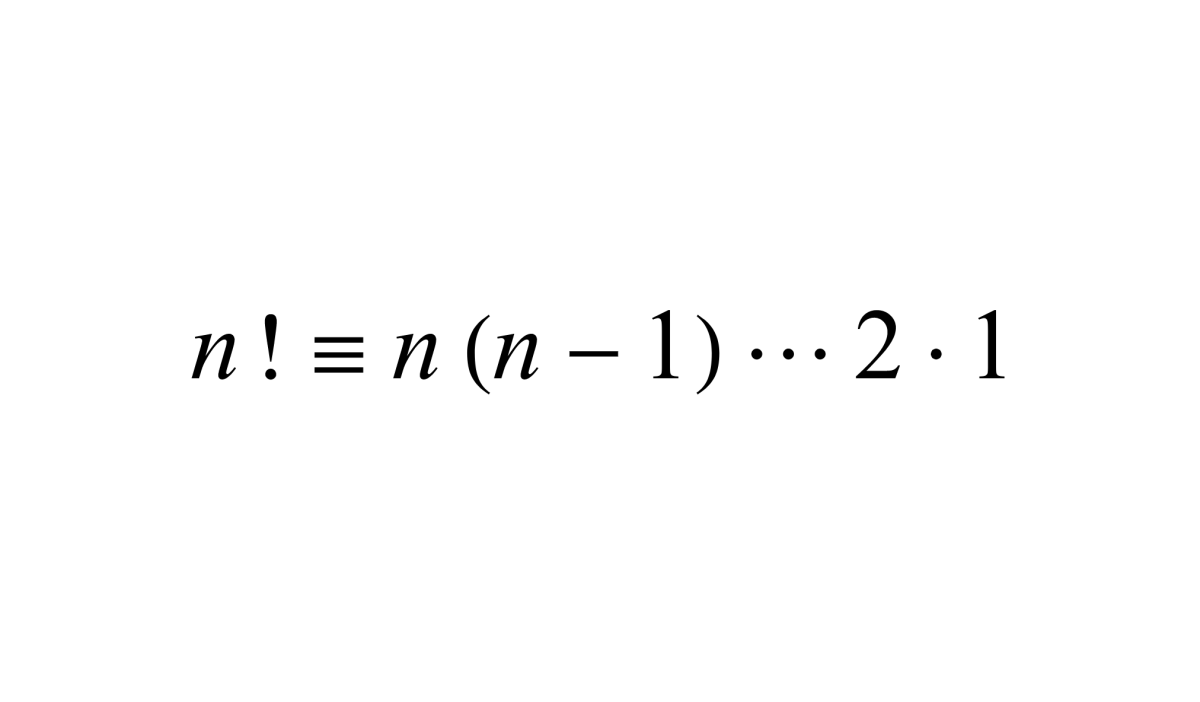# Find Factorial Using the FACT Function in Excel for Mac

Joshua earned an MBA from USF and he writes mostly about software and technology.How to use the FACT function in Excel to easily find the factorial of a number.

## The Purpose of the FACT Function

The FACT function gives an Excel user the ability to find the factorial of a number. To remind you of what a factorial is take for instance the factorial of 5 or 5!. The FACT function would calculate the factorial of 5 as 120 (1 × 2 × 3 × 4 × 5).

## The FACT Function Syntax

The FACT function syntax calls for the function to be inputted into a cell like a formula. To manually add this function the following steps need to be taken:

1. A cell needs clicked, type "=FACT(".
2. After the open parenthesis, a number or a cell reference needs to be added.
3. The number or reference is followed by a closing parenthesis.
4. After the formula is created the formula can be entered.

Below in bold, the syntax of the FACT function is shown followed by an explanation.

=FACT(Number)

Number - A number or cell reference.

## Fact Function Examples

Below are some examples of the FACT function in action. Each situation is detailed below.

Example 1 - In the first example, the outcome of the factorial will appear.

Example 2 - In the next example, a negative number ends in error.

Example 3 - The factorial of 0 = 1. This can be proven with the following: Where n=1, using n! = n*(n-1)! and 1! = 1*0!

Example 4 - For the last example, text will result in an error.

## Inserting the FACT Function

Like all other functions, the FACT function can be inserted into a cell. Even though there is only one argument (number) inserting the function will allow you to enter different numbers in a field to see different results.

Scroll to Continue

To insert the FACT function, start off by clicking into a cell. Next, click on the formulas tab followed by clicking on the Math & Trig option.

## Formula Builder

After the formula builder appears, add a number or a cell reference and either click done to insert the function or keep adding different arguments to view different results.

How to Use the COUNT Function in Excel

How to Use the AVERAGE Function in Excel

How to Use the ABS Function in Excel

How to Remove Errors in Excel With the IFERROR Function

How to Use the VLOOKUP Function in Excel

Converting Measurement Units: Using the CONVERT Function

Microsoft. (n.d.). FACT function. Retrieved August 5, 2022, from https://support.microsoft.com/en-us/office/fact-function-ca8588c2-15f2-41c0-8e8c-c11bd471a4f3

Britannica. (n.d.). Factorial. Retrieved August 5, 2022, from https://www.britannica.com/science/factorial

To learn more about using functions in Excel I recommend the Excel 2019 Bible book. I have been using the Excel Bible for years to improve my understanding of all aspects of this Microsoft product.

This content is accurate and true to the best of the author’s knowledge and is not meant to substitute for formal and individualized advice from a qualified professional.

© 2022 Joshua Crowder